Courses

# Test: Methods of Indeterminate Analysis -1

## 10 Questions MCQ Test Structural Analysis | Test: Methods of Indeterminate Analysis -1

Description
This mock test of Test: Methods of Indeterminate Analysis -1 for Civil Engineering (CE) helps you for every Civil Engineering (CE) entrance exam. This contains 10 Multiple Choice Questions for Civil Engineering (CE) Test: Methods of Indeterminate Analysis -1 (mcq) to study with solutions a complete question bank. The solved questions answers in this Test: Methods of Indeterminate Analysis -1 quiz give you a good mix of easy questions and tough questions. Civil Engineering (CE) students definitely take this Test: Methods of Indeterminate Analysis -1 exercise for a better result in the exam. You can find other Test: Methods of Indeterminate Analysis -1 extra questions, long questions & short questions for Civil Engineering (CE) on EduRev as well by searching above.
QUESTION: 1

### The following methods are used for structural analysis: 1. Macaulay method 2. Column analogy method 3. Kani’s method 4. Method of sections Those used for indeterminate structural analysis would include

Solution:

Macaulay's method is used for deflection and slope calculation due to point loads in prismatic beams. Method of sections is used for statically determinate trusses.

QUESTION: 2

Solution:
QUESTION: 3

### A loaded porta! frame is shown in figure. The profile of its Bending Moment diagram will be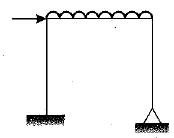Solution:

- The horizontal force will be distributed to both the supports.
- The bending moment at hinged end will be zero and fixed end will have some B.M.
- The B.M.D. for columns will be linear.
- The B.M.D. for beam will be parabolic.
The choice now remains between (b) and (d). In diagram (b) and (c) the Left column has negative B.M. in upper half or at top. The beam at left end in (b) shows negative B.M. while in (c) it shows a positive B.M.

QUESTION: 4

The given figure shows a portal frame with one end fixed and other hinged. The ratio of the fixed end moments MBA/MCD due to side sway will be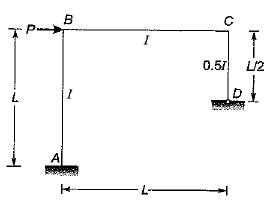Solution:

Due to sway, the deflection of point B will be equal to that of point C.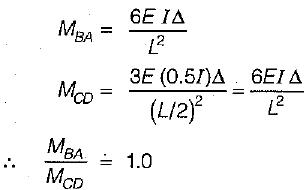QUESTION: 5

The distribution factors for members CB, CD and CG for the frame shown in the figure ( EI constant) will be respectively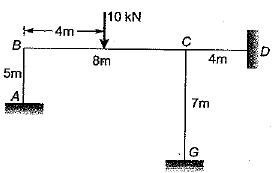Solution: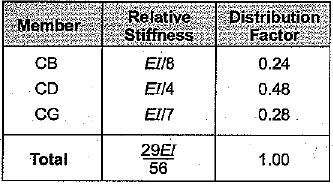QUESTION: 6

A fixed beam AB is subjected to a triangular load varying from zero at end A to w per unit length at end B. The ratio of fixed end moment at B to A will be

Solution: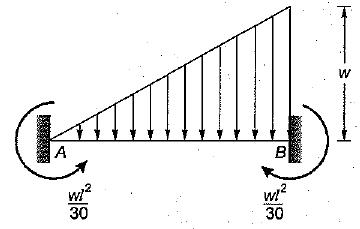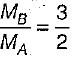QUESTION: 7

The fixed end moment MA for the beam shown in the given figure is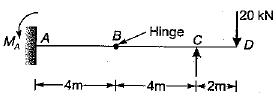Solution:

For part BD.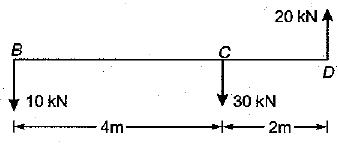For Part AB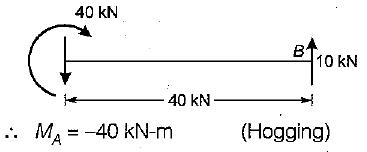QUESTION: 8

If the flexural rigidity of the beam BC of the portal frame shown in the given figure is assumed to be zero, then the horizontal displacement of the beam would be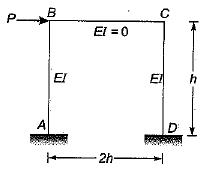Solution:

As the beam BC has no rigidity, so end B of column behaves as free end.
For a cantilever beam subjected to concentrated load at free end, the stiffness is 3EI/L3
Stiffness of given system,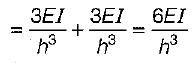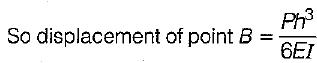QUESTION: 9

A portal frame is shown in the given figure. If θB - θc = 400/EI radian, then the value of moment at B will be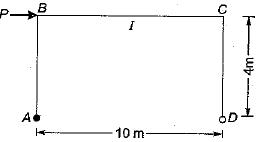Solution:

The deformed shape of structure will have translation at B and C,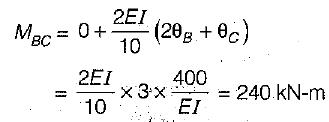QUESTION: 10

For the frame as shown in the figure below, the final end moment MBC has been calculated as -40 kN-m. What is the end moment MCD?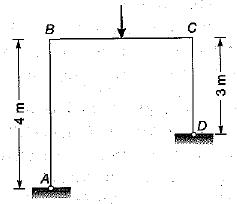Solution:

The shear force at end A should be equal and opposite to shear force at D, Let -40 kN-m denotes the clockwise moment so moment at end B of column AB is 40 kN-m anticiockwise and the shear force at A is10 kN towards left.
Therefore shear force at D is 10 kN towards right. Thus end moment at C is 30 kN-m clockwise or -30 kN-m.